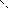# MINLPLib

### A Library of Mixed-Integer and Continuous Nonlinear Programming Instances

#### Instance inscribedsquare03

```The inscribed square problem, also known as the square peg problem or the Toeplitz' conjecture, is an unsolved question in geometry: Does every plane simple closed curve contain all four vertices of some square?
This is true if the curve is convex or piecewise smooth and in other special cases.
The problem was proposed by Otto Toeplitz in 1911. See also https://en.wikipedia.org/wiki/Inscribed_square_problem
This instance computes a maximal inscribing square for the curve (cos(t-t*t)*sin(t)-t*t*sin(2*t+3*abs(t)), sin(t)*t+0.5*t*t*cos(t)), t in [-pi,pi].```
 Formatsⓘ ams gms mod nl osil py Primal Bounds (infeas ≤ 1e-08)ⓘ 23.84938133 p1 ( gdx sol ) (infeas: 7e-09) Other points (infeas > 1e-08)ⓘ Dual Boundsⓘ 23.84952827 (COUENNE)23.84938219 (LINDO)23.84939367 (SCIP) Referencesⓘ Toeplitz, Otto, Über einige Aufgaben der Analysis situs, Verhandlungen der Schweizerischen Naturforschenden Gesellschaft, 94, 1911, 197. Sourceⓘ Benjamin Müller and Felipe Serrano Applicationⓘ Geometry Added to libraryⓘ 25 Sep 2019 Problem typeⓘ NLP #Variablesⓘ 8 #Binary Variablesⓘ 0 #Integer Variablesⓘ 0 #Nonlinear Variablesⓘ 6 #Nonlinear Binary Variablesⓘ 0 #Nonlinear Integer Variablesⓘ 0 Objective Senseⓘ max Objective typeⓘ quadratic Objective curvatureⓘ convex #Nonzeros in Objectiveⓘ 2 #Nonlinear Nonzeros in Objectiveⓘ 2 #Constraintsⓘ 8 #Linear Constraintsⓘ 0 #Quadratic Constraintsⓘ 0 #Polynomial Constraintsⓘ 0 #Signomial Constraintsⓘ 0 #General Nonlinear Constraintsⓘ 8 Operands in Gen. Nonlin. Functionsⓘ abs cos mul sin Constraints curvatureⓘ indefinite #Nonzeros in Jacobianⓘ 24 #Nonlinear Nonzeros in Jacobianⓘ 8 #Nonzeros in (Upper-Left) Hessian of Lagrangianⓘ 6 #Nonzeros in Diagonal of Hessian of Lagrangianⓘ 6 #Blocks in Hessian of Lagrangianⓘ 6 Minimal blocksize in Hessian of Lagrangianⓘ 1 Maximal blocksize in Hessian of Lagrangianⓘ 1 Average blocksize in Hessian of Lagrangianⓘ 1.0 #Semicontinuitiesⓘ 0 #Nonlinear Semicontinuitiesⓘ 0 #SOS type 1ⓘ 0 #SOS type 2ⓘ 0 Minimal coefficientⓘ 5.0000e-01 Maximal coefficientⓘ 3.0000e+00 Infeasibility of initial pointⓘ 5.506 Sparsity JacobianⓘSparsity Hessian of Lagrangianⓘ```\$offlisting
*
*  Equation counts
*      Total        E        G        L        N        X        C        B
*          9        9        0        0        0        0        0        0
*
*  Variable counts
*                   x        b        i      s1s      s2s       sc       si
*      Total     cont   binary  integer     sos1     sos2    scont     sint
*          9        9        0        0        0        0        0        0
*  FX      0
*
*  Nonzero counts
*      Total    const       NL      DLL
*         27       17       10        0
*
*  Solve m using DNLP maximizing objvar;

Variables  objvar,x2,x3,x4,x5,x6,x7,x8,x9;

Positive Variables  x8,x9;

Equations  e1,e2,e3,e4,e5,e6,e7,e8,e9;

e1.. -(sqr(x8) + sqr(x9)) + objvar =E= 0;

e2.. cos(x2 - x2*x2)*sin(x2) - x2*x2*sin(3*abs(x2) + 2*x2) - x6 =E= 0;

e3.. sin(x2)*x2 + 0.5*x2*x2*cos(x2) - x7 =E= 0;

e4.. cos(x3 - x3*x3)*sin(x3) - x3*x3*sin(3*abs(x3) + 2*x3) - x6 - x8 =E= 0;

e5.. sin(x3)*x3 + 0.5*x3*x3*cos(x3) - x7 - x9 =E= 0;

e6.. cos(x4 - x4*x4)*sin(x4) - x4*x4*sin(3*abs(x4) + 2*x4) - x6 + x9 =E= 0;

e7.. sin(x4)*x4 + 0.5*x4*x4*cos(x4) - x7 - x8 =E= 0;

e8.. cos(x5 - x5*x5)*sin(x5) - x5*x5*sin(3*abs(x5) + 2*x5) - x6 - x8 + x9 =E= 0
;

e9.. sin(x5)*x5 + 0.5*x5*x5*cos(x5) - x7 - x8 - x9 =E= 0;

* set non-default bounds
x2.lo = -3.14159265358979; x2.up = 3.14159265358979;
x3.lo = -3.14159265358979; x3.up = 3.14159265358979;
x4.lo = -3.14159265358979; x4.up = 3.14159265358979;
x5.lo = -3.14159265358979; x5.up = 3.14159265358979;

* set non-default levels
x2.l = -3.14159265358979;
x3.l = -1.5707963267949;
x5.l = 1.5707963267949;
x6.l = -1.31922501777732E-15;
x7.l = -4.93480220054468;
x8.l = 1;
x9.l = 1;

Model m / all /;

m.limrow=0; m.limcol=0;
m.tolproj=0.0;

\$if NOT '%gams.u1%' == '' \$include '%gams.u1%'

\$if not set DNLP \$set DNLP DNLP
Solve m using %DNLP% maximizing objvar;

```

Last updated: 2023-08-16 Git hash: 2519540e